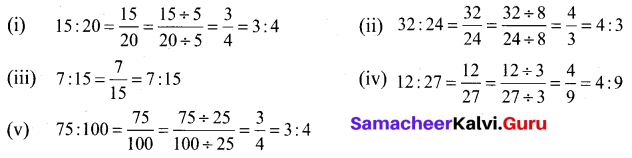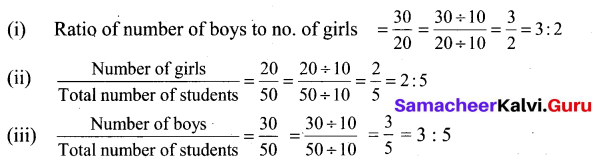Tamilnadu Samacheer Kalvi 6th Maths Solutions Term 1 Chapter 3 Ratio and Proportion Ex 3.1Question 1.
Fill in the blanks.
(i) Ratio of ₹ 3 to ₹ 5 = ____
(ii) Ratio of 3 m to 200 cm = ______
(iii) Ratio of 5 km 400 m to 6 km = ____
(iv) Ratio of 75 paise to ₹ 2 = ____
Solution:
(i) 3 : 5
(ii) 3 : 2
Hint: 3m = 300 cm
(iii) 9 : 10
Hint: 5km 400 m = 5400m and 6 km = 6000 m
(iv) 3 : 8
Hint: ₹ 2 = 200 paise

Question 2.
Say whether the following statements are True or False.
(i) The ratio of 130 cm to 1 m is 13 : 10.
(ii) One of the terms in a ratio cannot be 1.
Solution:
(i) True
(ii) False

Question 3.
Find the simplified form of the following ratios.
(i) 15 : 20
(ii) 32 : 24
(iii) 7 : 15
(iv) 12 : 27
(v) 75 : 100
Solution:Question 4.
Akilan walks 10 km in an hour while Selvi walks 6 km in an hour. Find the simplest ratio of the distance covered by Akilan to that of Selvi.
Solution:
Ratio of the distance covered by Akilan to that of Selvi = 10 km : 6 km
= $$\frac{10}{6}$$
= $$\frac{5}{3}$$
= 5 : 3

Question 5.
The cost of parking a bicycle is ₹ 5 and the cost of parking a scooter is ₹ 15. Find the simplest ratio of the parking cost of a bicycle to that of a scooter.
Solution:
Parking cost of bicycle = ₹ 5
Parking cost of Scooter = ₹ 15
$$\frac{\text { Parking cost of bicyle }}{\text { Parking cost of scooter }}=\frac{5}{15}=\frac{1}{3}$$
Parking cost of bicycle : scooter = 1 : 3Question 6.
Out of 50 students in class 30 are boys. Find the ratio of
(i) number of boys to the number of girls
(ii) number of girls to the total number of students
(iii) number of boys to the total number of students
Solution:
The total number of students = 50.
The number of boys = 30.
Then number of girls = 50 – 30 = 20.Objective Type Questions

Question 7.
The ratio of Rs 1 to 20 paise is
(a) 1 : 5
(b) 1 : 2
(c) 2 : 1
(d) 5 : 1
Solution:
(d) 5 : 1

Question 8.
The ratio of 1 m to 50 cm is _____
(a) 1 : 50
(b) 50 : 1
(c) 2 : 1
(d) 1 : 2
Solution:
(c) 2 : 1
Hint: 1 m = 100 cm

Question 9.
The length and breadth of a window are in 1 m and 70 cm respectively. The ratio of the length to the breadth is
a) 1 : 7
(b) 7 : 1
(c) 7 : 10
(d) 10 : 7
Solution:
(d) 10 : 7

Question 10.
The ratio of the number of sides of a triangle to the number of sides of a rectangle is ____
(a) 4 : 3
(b) 3 : 4
(c) 3 : 5
(d) 3 : 2
Solution:
(b) 3 : 4
Triangle has three sides and a rectangle has four sides.Question 11.
If Azhagan is 50 years old and his son is 10 years old then the simplest ratio between the age of Azhagan to his son is
(a) 10 : 50
(b) 50 : 10
(c) 5 : 1
(d) 1 : 5
Solution:
(c) 5 : 1